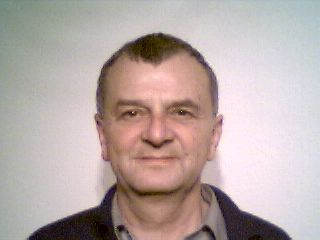## Fourier TransformLet me know
what you thinkFourier SeriesResonatorsWhy Cosine and Sine ?Physical and Mathematical DictionsDiscrete Fourier Transform DFTOptimized DFT Sample ProgramDiscrete Cosine Transform DCTAn Example of DCT from Victor Lo with N=8## Fourier Series

Jean Baptiste Joseph Baron de Fourier (1768-1830) in: Théorie analytique de la chaleur, Paris 1822 derived this law:
Any periodic function f(x) can be approximated by the sum of a constant plus a series of cosine- and sine-waves: the so-called Fourier series:Please notice: The summation index k occurs as frequency multiplier in both arguments.
Arbitrary periodic functions can be expressed in form of such a series.

The simplified Fourier series is composed of:

1. A constant a0/2, where a0 is the average of the function f(x).
2. When the periodic function has a period T, then we have to add a cosine and sine function which both have a period T (1/T = basic frequency) with the still to be computed amplitudes a1 for the cosine and b1 for the sine: f(x) = a0/2 +a1*cos(x) + b1*sin(x).
3. It follow a cosine and a sine with half the period =T/2 and the (still to be computed) amplitudes a2 and b2:
f(x) = a0/2 +a1*cos(x) + b1*sin(x) + a2*cos(2*x) + b2*sin(2*x).
4. again another two terms with the period =T/3 and the (still to be computed) amplitudes a3 and b3 and so on further pairs of cosines and sines, with T divided by integers T/4, T/5 ...
The denominator of T appears as a k multiplier, because the division of T by k is equivalent to multiplying the frequency: x*2*Pi/(T/k) = k*x*2*Pi/T.

A further introduction can be found here: http://en.wikipedia.org/wiki/Fourier_transform

## Resonators

The physicist Fourier was inspired by physical experiments:
It's no problem to produce ugly and dissonant sounds with string instruments even so the strings can just vibrate in their harmonics: Any mixture of pure frequencies normally produces dissonances and only seldom sounds.
Fourier's intuitive and unproven reverse conclusion was, that any arbitrary noise can be produced by a summation of pure wave components. This intuitive conclusion turned out to be an extraordinary scientific insight.

Example 1: In the cochlea of the inner ear exists a fluid-mechanical system with harmonic residence points carrying sensory cells. These hair cells with motion sensitive hairs = cilia measure the displacement of the resonator membrane. The electric surface potential of the hair cells changes instantaneously and synchronous to this displacement. This is similar to the voltage change coming out from a microphone with responds to the movement of the microphone membrane. The hair cells release neurotransmitters into the extracellular space, which trigger nerve impulses in exactly one fiber of the secondary neuron, now carrying information about the amplitude of one specific characteristic frequency.

Example 2: An electric circuit of a coil and a parallel capacitor has a specific resonant frequency. It recognizes the presence or absence of a single frequency in any mixture and gets excited whenever its frequency exists somewhere inside the mix. There is no active process, it's merely a passive be forced into resonance.
These resonant circuits are the basis of electrical audio technology. An ordered set of resonant circuits is exposed to an arbitrary signal and only the frequencies fitting a resonator are detected. These output signals from the different resonators are amplified and mixed again and the result is an identical but amplified signal, which is only composed of the few pure frequencies coming out of the resonators.
It's an astonishing experience that relatively few resonators are sufficient to amplify arbitrary waves.

Example 3: A pendulum only swings in one specific frequency. When you wish to increase the amplitude of a child on the swing, you must push the swing at its resonant frequency. It's impossible to increase the amplitude by pushing continuously or with any other frequency.

Summary: Fourier transformation is the splitting of a periodic oscillation into a finite or infinite sum of harmonic oscillations of multiples of a fundamental frequency and the reversal of this decomposition, which means the reconstruction of an arbitrary waveform through addition of harmonic oscillations.

The sines and cosines of the fourier series can be regarded as mathematical resonators in analogy to electrical resonators with the ability to detect the presence of specific frequencies in a mixture and when detected to quantify their contributions to the overall mixed signal = their relative amplitudes (relative to the half of the average amplitude of the mixture).

For the sake of simplicity we stretch `T` to the length of `2*Pi`.
Joseph Fouriers genial idea is the following:
(1) He multiplies the original signal `f(x)` with the pure resonators `cos(k*x)` and `sin(k*x)`. For every `k` he obtains two product signals `f(x)*cos(k*x)` and `f(x)*sin((k*x)` with the following properties: The products always have positive and negative values, even in case when the original `f(x)` was just positive or just negative. The products are highly positive where a mountain meets a mountain or where a valley meets a valley (=case 1) and highly negative where a mountain meets a valley (=case 2).
When case 1 occurs as often as case 2 in `f(x)*cos(k*x)` or `f(x)*sin(k*x)`, the sum is `0` and `f(x)` has nothing to do with `cos(k*x)` or `sin(k*x)`.
When case 1 occurs more often than case 2 in `f(x)*cos(k*x)` or `f(x)*sin(k*x)`, the sum is positive and `f(x)` and `cos(k*x)` or `sin(k*x)` have the common frequency no. `k`.
When case 2 occurs more often than case 1 in `f(x)*cos(k*x)` or `f(x)*sin(k*x)`, the sum is negative and `f(x)` and `cos(k*x)` or `sin(k*x)` have the common frequency no. `k`.

(2) With these cases in mind Fourier uses the sums of the product functions as resonators. The sums are computed as integrals of `f(x)*cos(k*x)*dx` and `f(x)*sin(k*x)*dx` over the period `T`.
Dividing the integrals by `T` furnishes the normalized resonance answers, the so called Fourier coefficients: two answers `ak` and `bk` for each resonance test with frequency no. `k`: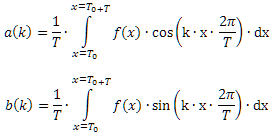## Example

 `f(x)` is composed of 3 sinus curves and noise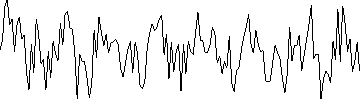`ak2`+`bk2 `(with` 0 <= k < N`)## Why Cosine and Sine ?

Why does Fourier need two resonators (cosine und sine) per test frequency ? Why cosine or sine alone will not do ?
Answer: When the peek of a mountain of `f(x)` meets a zero point of `cos(k*x)`, the product has a positive and a negative half which sum up to `0` even if the mountain has the same size and extent as `cos(k*x)`.
For this case Fourier needs a twin resonator of the same frequency but 90 degrees shifted in phase to be sure that at least one of the twins comes into resonance.
Electrical and other physical resonators do not need such twins. Without excitation they stay at rest, they do not oscillate at their own. On excitation they come slowly into resonance in phase with the signal they receive.
In contrary the mathematical Fourier resonators always oscillate at their own without any sensibility to the outside world and without any ability to synchronize with it. They have the character of permanent test waves forming products and product integrals with possible real `f(x)` waves and you need two of them for any harmonic frequency to be sure that at least one will come into resonance when there is something in `f(x)` which fits.
We will see later that when you force `f(x)` into a fixed phase position symmetrically to `x=0` actually one Fourier resonator will be sufficient -→ Cosine Transform.

Summary: Fourier builds, starting with the base frequency `2*Pi/T`, two resonators for any integer frequency multiplier and tries out any of these resonators. The test is the multiplication of resonator and signal and integration of the results. If positive and negative areas compensate to `0` the test is negative, otherwise positive. This procedure is far from being elegant or effectif, it is a brute force strategy. It needs excessive computation power, a hopeless thing in times without computers, where nobody was able to demonstrate the transform.

## Physical and Mathematical Dictions

In the tradition of 19. century we spoke in terms of physics because it's far more simple and intuitive than the abstract and generalized mathematical diction.

Analogy of harmonic composition = harmonic synthesis:
Physics: superposition = It's possible to compose any arbitrary waveform by superpositioning pure waves.
Mathematics: sum = It's possible to approximate any periodic function by sums of cosine- and sine-functions.

Analogy of integers:
Physics: Any oscillation can be built up from a basic frequency and integer multiples of it = harmonic waves.
Example: A violin string clamped between to points can only swing in integer multiples of its basic frequency because no movement is possible at the clamping points. It's impossible that the oscillation is composed by non-integer multiples of the basic frequency (for example 2,2 or 7,5) because such oscillations would tear the string from one clamping point.
Mathematics: Any arbitrary periodic function `f(x)` is a point in an orthogonal normalized vector space, with the orthogonal basis vectors `cos(k)` and `sin(k)`.

Analogy of harmonic decomposition = harmonic analysis:
Physics: Each oscillation can be divided by resonators into its components. As many resonators as pure waves are needed and the resonators must be narrow banded integer multiples of the basic frequency.
Mathematics: The Fourier transform is the convolution of `f[x]` with `cosinus(k*arg)` and `sinus(k*arg)` for any integer multiple of the argument `arg` furnishing the orthogonal coordinates `ak` and `bk`.

further analogies:

 physical diction mathematical diction time `t` independent variable `x` elongation(`t`), sound pressure(`t`), field strength(`t`) function `y = f(x)` basic wave length `T` argument multiplier `L = 2*Pi/T` harmonics with wave lengths `T/2, T/3, T/4` etc. rescalers `2, 3, 4` etc. of the arguments resonator of the `k`th harmonic basis vectors `cos(k*L)` and `sin(k*L)` `ak` and `bk` are the resonance answers of both `k`th resonators `ak` and `bk` = results of the convolution normal position constant `a0/2` amplitude of the base oscillation square root of `(a1*a1 + b1*b1)` amplitude of the `k`th harmonic square root of `(ak*ak + bk*bk)`

## Discrete Fourier Transform DFT

Functions are stored in computers mainly as discrete equidistant data in form of one-dimensional arrays (for example fever curves, stock exchange rates, pixels in rows and columns).
Regardless if such data have periodicity the Fourier transform is very useful to remove unwanted artifacts and to lower redundancy = compression. Joseph Fourier never thought of such things, but today they are the most popular applications of his harmonic analysis.
The computation of `ak` and `bk` from discrete data follows the Fourier rules:
Given a signal `f(x)` in form of an array of length `N`
`N` takes the role of period `T` and each resonator takes the form of an array of length `N`.
Sums from `0` till `N-1` replace the integrals from `T0` till `T0+T`.Since we need `N` cosine- and `N` sine-resonators, we prepare the resonators in a suitable way in form of a quadratic `N*N`-cosine-matrix and a sine-matrix of the same size.
Multiplication and summation have now the form of scalar multiplication of `f(x)` with all rows of both matrices.
The `ak` und `bk` are computed from the `N + N` scalar products: `f(x)` multiplied with one row of one matrix divided by `N`. The amount of necessary floating point operations is enormous:
`N*N` computations of cosine plus
`N*N` computations of sinus plus
`2*N*N` multiplications plus
`2*N*N` additions plus
`2*N` divisions.

 Example of a Fourier decomposition of a signal with `N=4`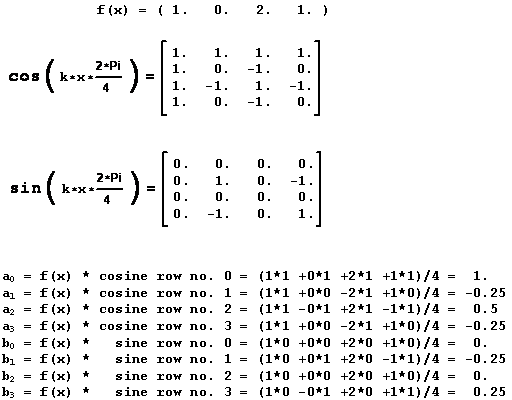```//C# program for this `N=4` example
using System;
using System.Drawing;
using System.Windows.Forms;
using System.Text;

public class Form1 : Form
{ [STAThread] static void Main() { Application.Run( new Form1() ); }
const int N = 4;
float[] f0 = { 1f, 0f, 2f, 1f }, f1  = new float[N];
float[,] cos = new float[N,N]  , sin = new float[N,N];
float[]  a   = new float[N]    , b   = new float[N];
StringBuilder sb = new StringBuilder();
public Form1()
{ Text = "Prof. Miszalok's Fourier sample with N=4";
Width = 320; Height = 400;
int x, k;
for ( k=0; k < N; k++ ) //prepare resonators
for ( x=0; x < N; x++ )
{ cos[k,x] = Convert.ToSingle( Math.Cos( k*x*Math.PI/2.0 ) ) ;
sin[k,x] = Convert.ToSingle( Math.Sin( k*x*Math.PI/2.0 ) ) ;
}
for ( k=0; k < N; k++ ) //Fourier transform
{ for ( x=0; x < N; x++ )
{ a[k] += f0[x] * cos[k,x]; //convolution
b[k] += f0[x] * sin[k,x]; //convolution
}
a[k] /= N; b[k] /= N; //normalization
}
for ( k=0; k < N; k++ ) //back transform
for ( x=0; x < N; x++ ) { f1[x] += a[k]*cos[k,x] + b[k]*sin[k,x]; }
//compose output string
sb.Append ("f0:\r\n");
for ( x=0; x < N; x++ )   sb.Append( String.Format( "{0,5:F0}", f0[x]) );
sb.Append( "\r\ncos:\r\n" );
for ( k=0; k < N; k++ )
{ for ( x=0; x < N; x++ ) sb.Append( String.Format( "{0,5:F0}", cos[k,x]) );
sb.Append( "\r\n" );
}
sb.Append( "sin:\r\n" );
for ( k=0; k < N; k++ )
{ for ( x=0; x < N; x++ ) sb.Append( String.Format( "{0,5:F0}", sin[k,x]) );
sb.Append( "\r\n" );
}
sb.Append( "a:\r\n" );
for ( k=0; k < N; k++ )   sb.Append( String.Format( "{0,9:F2}", a[k] ) + "\r\n" );
sb.Append( "b:\r\n" );
for ( k=0; k < N; k++ )   sb.Append( String.Format( "{0,9:F2}", b[k] ) + "\r\n" );
sb.Append( "f1:\r\n" );
for ( x=0; x < N; x++ )   sb.Append( String.Format( "{0,5:F0}", f1[x]) );
sb.Append( "\r\n" );
}
protected override void OnPaint( PaintEventArgs e )
{  e.Graphics.DrawString( sb.ToString(), Font, new SolidBrush( Color.Red ), 0, 0 ); }
}```

## Optimized DFT Sample Program

The following C#-program
1) fills an array `f0` with 3 frequencies,
2) computes the `ak` and the `bk`,
3) transforms them back into an array `f1`,
4) makes an output string and displays it,
5) reduces memory to 50% and computing time to 30% compared with the raw Fourier formula.
The program derives advantage from inherent Fourier symmetry and limits (without any loss of precision) the no.s of `ak` and `bk` to `N/2+1`. Reason: The original `f[x]` has `N` elements and when both the `ak` and the `bk` have `N` elements, there must be a redundancy of 50%. Indeed, the high numbered `ak` and `bk` with `k > N/2` mirror the low numbered with `k < N/2` in the following way:
1) when `N` is even: `a0`,...,`aN/2`, `aN/2-1`, `aN/2-2`,...,`a1`. Sample `N=8`: a,b,c,d,e,d,c,b
2) when `N` is odd : `a0`,...,`aN/2`, `aN/2`, `aN/2-1`, `aN/2-2`,...,`a1`. Sample `N=9`: a,b,c,d,e,e,d,c,b
Conclusion: There is no need to compute more than `N/2+1` `ak` and `bk`. It follows that the `cos[,]` and `sin[,]` resonator matrices don't need to be quadratic: `N/2+1` rows (with `N` columns each) are sufficient.
There is another interesting symmetry inside the remaining resonator matrices: The upper right half is identical to the lower left half with diagonal symmetry because `cos(k*x*arcus)` is identical with `cos(x*k*arcus)` and same with `sin(...)`. Therefore it's possible to compute the upper right corner and to mirror the values to down left.
Further accelerations:
1) We set the first row and column of `cos(k*x*arcus)` always to `1f` and of `sin(k*x*arcus)` always to `0f` without any computation.
2) We set `a0` always to the average of `f[x]` and `b0` always to `0f` without any convolution.
The disadvantages of these accelerations are:
1) The code is more complex than before.
2) The normalization becomes tricky compared to the Fourier formula: We have to double all `ak` and `bk` except `a0`,`b0` and `aN/2`,`bN/2`.
Summary: An optimized Fourier transform can be surprisingly fast. In cases with `N < 1000` there is no need to use any Fast Fourier Transform FFT with its multiple restrictions and inaccuracies.

```using System;
using System.Drawing;
using System.Windows.Forms;
using System.Text;

public class Form1 : Form
{ [STAThread] static void Main() { Application.Run( new Form1() ); }
const int N = 16;
float[]  f0  = new float[N]      , f1  = new float[N];      //input, output
float[,] cos = new float[N/2+1,N], sin = new float[N/2+1,N];//resonators
float[]  a   = new float[N/2+1]  , b   = new float[N/2+1];  //Fourier coefficients
StringBuilder sb = new StringBuilder();                     //one string takes all
public Form1()
{ Text = "Prof. Miszalok's Fourier sample with N=16";
Width = 500; Height = 600;
int x, k;
double arcus = 2*Math.PI/N, k_arcus, x_k_arcus;
for ( x=0; x < N; x++ ) //input = mixture of 3 frequencies, just for fun
{ f0[x]  = (float)Math.Cos(     x * arcus );
f0[x] += (float)Math.Sin( 3 * x * arcus );
f0[x] += (float)Math.Cos( 6 * x * arcus + Math.PI/4 );
}
//prepare resonators
for ( x=0; x <   N;  x++ ) { cos[0,x] = 1f; sin[0,x] = 0f; } //row no. 0
for ( k=0; k <= N/2; k++ ) { cos[k,0] = 1f; sin[k,0] = 0f; } //column no. 0
for ( k=1; k <= N/2; k++ ) //resonator rows 1 to N/2
{ k_arcus = k * arcus;
for ( x=k; x < N; x++ )  //upper right triangle of cos[,] and sin[,]
{ x_k_arcus = x * k_arcus;
cos[k,x] = Convert.ToSingle( Math.Cos( x_k_arcus ) ) ;
sin[k,x] = Convert.ToSingle( Math.Sin( x_k_arcus ) ) ;
if ( x <= N/2 && k != x )  //mirror to lower left except diagonal
{ cos[x,k] = cos[k,x]; sin[x,k] = sin[k,x]; }
}
}
for ( x=0; x < N; x ++ ) a += f0[x]; a /= N; b = 0f;
for ( k=1; k <= N/2; k++ ) //Fourier transform
{ for ( x=0; x < N; x++ )
{ a[k] += f0[x] * cos[k,x]; //convolution
b[k] += f0[x] * sin[k,x]; //convolution
}
if ( k < N/2 ) { a[k] *= 2f/N; b[k] *= 2f/N; } //doubling and normalization
else           { a[k] /= N;    b[k] /= N;    } //normalization
}
for ( k=0; k <= N/2; k++ ) //back transform
for ( x=0; x < N; x++ ) { f1[x] += a[k]*cos[k,x] + b[k]*sin[k,x]; }
//compose output string
sb.Append ("f0:\r\n");
for ( x=0; x < N; x++ )   sb.Append( String.Format( "{0,6:F1}", f0[x]) );
sb.Append( "\r\ncos:\r\n" );
for ( k=0; k <= N/2; k++ )
{ for ( x=0; x < N; x++ ) sb.Append( String.Format( "{0,6:F1}", cos[k,x]) );
sb.Append( "\r\n" );
}
sb.Append( "sin:\r\n" );
for ( k=0; k <= N/2; k++ )
{ for ( x=0; x < N; x++ ) sb.Append( String.Format( "{0,6:F1}", sin[k,x]) );
sb.Append( "\r\n" );
}
sb.Append( "a:\r\n" );
for ( k=0; k <=N/2; k++ ) sb.Append( String.Format( "{0,9:F2}", a[k] ) + "\r\n" );
sb.Append( "b:\r\n" );
for ( k=0; k <=N/2; k++ ) sb.Append( String.Format( "{0,9:F2}", b[k] ) + "\r\n" );
sb.Append( "f1:\r\n" );
for ( x=0; x < N; x++ )   sb.Append( String.Format( "{0,6:F1}", f1[x]) );
sb.Append( "\r\n" );
}
protected override void OnPaint( PaintEventArgs e )
{ e.Graphics.DrawString( sb.ToString(), Font, new SolidBrush( Color.Red ), 0, 0 ); }
}```

Experiments:
1. Try out the back transform with frequency cuts by replacing the upper limit `N/2` of the back transform loop successively by `0,1,2...` till `7` and observe how `f1[x]` comes closer to `f0[x]`.
2. Explain the positions and the signs of the values `ak != 0f` and `bk != 0f`.
3. Mix new and higher frequencies into `f0` by inserting new lines `f0[i] += (float)Math....( ... );` into the first `for`-loop of the program.
4. Mix 10% noise to `f0`:
```    Random r = new Random();     f0[i] += 0.1f * (float)( r.NextDouble()-0.5 );```
and limit the back transform to `k <= 7`.
5. Increase `N` to `N > 20`;
6. You will find more programs dealing with Fourier transforms here:
`    `www.miszalok.de/C_CVCis/C6_FourierTransform = Analysis of contours in raster drawings
`    `www.miszalok.de/C_CVCis/C5_FourierRecognition = Learning Optical Character Recognition

## Discrete Cosine Transform DCT

We elongate the array `f(x)` by `N-1` elements and fill the elongation by symmetric mirroring at the mid of the last element `N-1`, which copies `N-2→N, N-3→N+1, N-4→N+2` etc. till `0→N+N-2`: We obtain an array of odd length `2*N-1`.
This array now contains an even function having its mirror axis at position `N-1+½`. All values with the same distance left and right from `N-1+½` are identical:
For all `0<=i<=N-2` we obtain `f(N-1-i) == f(N-1+i)`.
Now you have to take care that the cosine-resonators have a extremum at position `N-1+½` and that the sine-resonators have `0` at position `N-1+½`. In this case the `bk` always are `0`. The integrals of the products of `f(x)` with all sine-resonators vanish because sine is an odd function with symmetry at position `N-1+½` whereas cosine is an even function with line symmetry at position `N-1+½` as is the mirrored `f(x)`.
With other words, we just have to compute `ak`. Computing and memory costs remain the same because the drop of sine-resonators is compensated by the doubling of cosine-resonators.
The trick is to limit the amount of cosine-resonators to a very small number and to ignore the errors that follow. It became apparent that the DCT is remarkably tolerant and kind toward such shortenings. This is the reason why it is popular and the modern basis of many effective compression algorithms.
The first practical simplification is to deliberately forget the mirroring of `f(x)`. It's possible to compute everything from the original array length of `N` by stretching (by `2` to the right) and shifting (by `0.5` to the left) the cosine-resonators.` `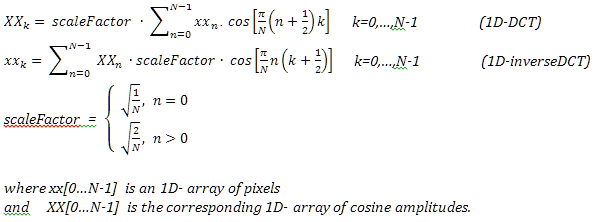The code length of the DCT algorithm is much shorter than the corresponding DFT code (but execution time remains identical):

```using System;
using System.Drawing;
using System.Windows.Forms;
using System.Text;

public class Form1 : Form
{ [STAThread] static void Main() { Application.Run( new Form1() ); }
const int N = 5;
float[] f0 = { 1f, 3f, -3f, 2f, 3f }, f1  = new float[N];
float[,] cos = new float[N,N];
float[]  a   = new float[N];
StringBuilder sb = new StringBuilder();
public Form1()
{ Text = "Prof. Miszalok's DCT sample with N=5";
Width = 320; Height = 400;
int x, k;
for ( k=0; k < N; k++ ) //prepare resonators slowly
for ( x=0; x < N; x++ ) cos[k,x] = Convert.ToSingle( Math.Cos( (x+0.5)*(k+0.5)*Math.PI/N ) );
for ( k=0; k < N; k++ ) //Discrete Cosine Transform
{ for ( x=0; x < N; x++ ) a[k] += f0[x] * cos[k,x]; //convolution
a[k] *= 2f/N; //normalization
}
for ( k=0; k < N; k++ ) //back transform
for ( x=0; x < N; x++ ) f1[x] += a[k]*cos[k,x];
//compose output string
sb.Append ("f0:\r\n");
for ( x=0; x < N; x++ )   sb.Append( String.Format( "{0,5:F0}", f0[x]) );
sb.Append( "\r\ncos:\r\n" );
for ( k=0; k < N; k++ )
{ for ( x=0; x < N; x++ ) sb.Append( String.Format( "{0,5:F0}", cos[k,x]) );
sb.Append( "\r\n" );
}
sb.Append( "a:\r\n" );
for ( k=0; k < N; k++ )   sb.Append( String.Format( "{0,9:F2}", a[k] ) + "\r\n" );
sb.Append( "f1:\r\n" );
for ( x=0; x < N; x++ )   sb.Append( String.Format( "{0,5:F0}", f1[x]) );
sb.Append( "\r\n" );
}
protected override void OnPaint( PaintEventArgs e )
{  e.Graphics.DrawString( sb.ToString(), Font, new SolidBrush( Color.Red ), 0, 0 ); }
}```

## An Example of DCT from Victor Lo with N=8

The following tables have been taken (and slightly changed) from: Victor Lo, City University of Hong Kong: "MPEG 2 Beginners Guide"

 Integration of `a0` and `a1` 8 cosine-resonators scanned 8 times in `T`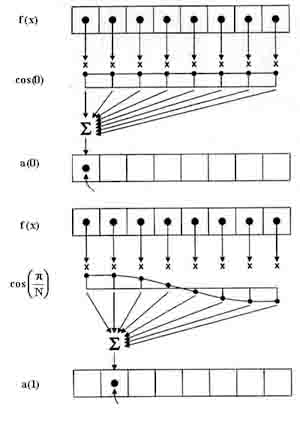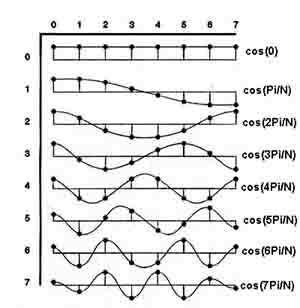Result\SampleIndex 0 1 2 3 4 5 6 7 0 0.707 0.707 0.707 0.707 0.707 0.707 0.707 0.707 1 0.981 0.831 0.556 0.195 -0.195 -0.556 -0.831 -0.981 2 0.924 -0.383 -0.383 -0.924 -0.924 -0.383 0.383 0.924 3 0.831 -0.195 -0.981 -0.556 0.556 0.981 0.195 -0.831 4 0.707 -0.707 -0.707 0.707 0.707 -0.707 -0.707 0.707 5 0.556 -0.981 0.195 0.831 -0.831 -0.195 0.981 -0.556 6 0.383 -0.924 0.924 -0.383 -0.383 0.924 -0.924 0.383 7 0.195 -0.556 0.831 -0.981 0.981 -0.831 0.556 -0.195